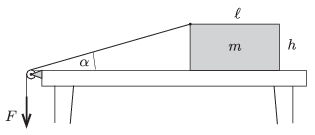Mathematical and Physical Journal
for High Schools
Issued by the MATFUND Foundation
 Already signed up? New to KöMaL?

#Problem P. 4804. (January 2016)

P. 4804. A uniform block of mass $\displaystyle m$ is pulled along the horizontal tabletop as shown in the figure. One end of the thread is attached to the midpoint of the top horizontal edge (perpendicular to the plane of the figure) of the block. The thread is led through a pulley and its other end is pulled by a force $\displaystyle F$, which is varied in order that the block moves at a constant speed. The coefficient of kinetic friction between the tabletop and the block is $\displaystyle \mu$.$\displaystyle a)$ Determine how the tension in the thread depends on the angle $\displaystyle \alpha$ shown in the figure.

$\displaystyle b)$ What can the maximum value of this angle $\displaystyle \alpha$ be while the block is sliding uniformly as prescribed above?

Data: $\displaystyle \ell=17.3$ cm, $\displaystyle h=11$ cm, $\displaystyle mg=10$ N, $\displaystyle \mu=0.15$.

(6 pont)

Deadline expired on February 10, 2016.

### Statistics:

 66 students sent a solution. 6 points: Balogh Menyhért, Bekes Nándor, Csorba Benjámin, Csuha Boglárka, Elek Péter, Fajszi Bulcsú, Fehér 169 Szilveszter, Forrai Botond, Jakus Balázs István, Juhász 326 Dániel, Kasza Bence, Krasznai Anna, Mány Bence, Marozsák Tóbiás , Németh 777 Róbert, Németh Flóra Boróka, Olosz Adél, Sal Kristóf, Szabó Ágoston, Szőke Dániel, Tomcsányi Gergely, Tóth Adrián. 5 points: Büki Máté, Kovács Péter Tamás, Simon Dániel Gábor. 4 points: 29 students. 3 points: 6 students. 2 points: 2 students. 1 point: 2 students. 0 point: 2 students.

Problems in Physics of KöMaL, January 2016